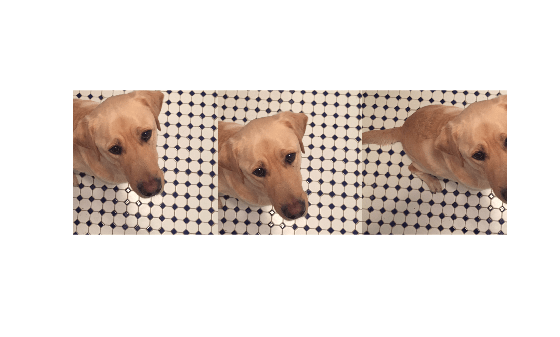# randomCropWindow2d

Create randomized rectangular cropping window

## Syntax

``win = randomCropWindow2d(inputSize,targetSize)``

## Description

example

````win = randomCropWindow2d(inputSize,targetSize)` determines the window to crop from a 2-D input image of size `inputSize` such that the size of the cropped image is `targetSize`. The coordinates of the window are selected from a random position in the input image.```

## Examples

collapse all

```A = imread('kobi.png'); imshow(A)```Specify the target size of the cropping window.

`targetSize = [1000 1000];`

Create three random crop windows. Each window has a different position from the input image.

```win1 = randomCropWindow2d(size(A),targetSize); win2 = randomCropWindow2d(size(A),targetSize); win3 = randomCropWindow2d(size(A),targetSize);```

Crop the original image using each of the random crop windows.

```B1 = imcrop(A,win1); B2 = imcrop(A,win2); B3 = imcrop(A,win3);```

Display the three cropped images as a montage.

`montage({B1,B2,B3},'Size',[1 3]);`## Input Arguments

collapse all

Input image size, specified as one of the following.

Type of Input ImageFormat of `inputSize`
2-D grayscale or binary image2-element vector of positive integers of the form ```[height width]```
2-D RGB or multispectral image of size 3-element vector of positive integers of the form ```[height width channels]```

Data Types: `single` | `double` | `int8` | `int16` | `int32` | `int64` | `uint8` | `uint16` | `uint32` | `uint64`

Target image size, specified as one of the following.

Type of Target ImageFormat of `targetSize`
2-D grayscale or binary image2-element vector of positive integers of the form ```[height width]```
2-D RGB or multispectral image of size 3-element vector of positive integers of the form ```[height width channels]```

Data Types: `single` | `double` | `int8` | `int16` | `int32` | `int64` | `uint8` | `uint16` | `uint32` | `uint64`

## Output Arguments

collapse all

Cropping window, returned as a `Rectangle` object.